# Mathematics 1010 online

## Complex Numbers

Recall how we built the number system : We started with the set of natural numbers, expanded it to the integers to make subtraction always possible, expanded them to rational numbers to make division always possible (except division by zero), and then expanded them to real numbers to account for the existence of numbers that manifestly are not rational.

We have to expand the numbers system one more time, to include complex numbers. Unfortunately it is not easy to motivate this expansion as compellingly as the previous expansions. Complex numbers have a tremendous range of applications, that will become apparent as you move on in Mathematics, Science, and Engineering, but they may be hard to appreciate at this stage.

For our purposes we start with the observation that there is no real numbersuch that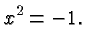This follows from the fact that the square of a positive number is positive, and that of a negative number is positive also. Thus there's no positive or negative number whose square is negative (and the square of zero of course is also non-negative).

We now take a leap of faith the kind of which sometimes gives mathematics a bad name. We don't know of a number satisfying the above equation, so we stipulate that there is a solution and we give it a name. We call it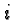.

There are only two things aboutwe need to know and appreciate. One is the fact that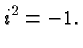The second fact is that we define the arithmetic operations withsuch that the ordinary rules of arithmetic continue to hold.

Let's consider some consequences of this fact. We obtain in particular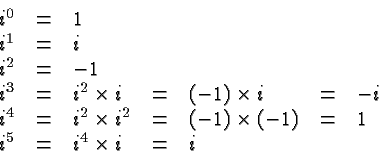It is clear how this process continues. The powers ofwith natural numbers as exponents areand so on forever. We also have, for example,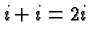,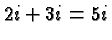,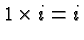,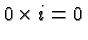, etc. What about the sum ofand a real number like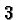? In other words, what is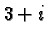? There is no way to simplify that expression, and so we leave it as it is!

Let's take stock and introduce some language. The symbolis called the imaginary unit. A complex number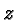is an expression that can be written in the form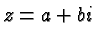whereand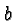are real numbers (andmultiplies). That particular form is sometimes called the standard form of a complex number.is called the real part of, andis its imaginary part. If the real part ofis zero, and the imaginary part non-zero, thenis called an imaginary number. (If the imaginary part is zero, thenis a real number.) If you want to emphasize that the real part is zero you can call the number pure imaginary or purely imaginary.

Note that the imaginary part of a complex number is in fact a real number (and not, as you might reasonably expect, an imaginary number).

Consider, for example, the complex number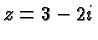. Its real part is, and its imaginary part is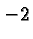. The number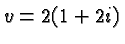is also a complex number. Its standard form is, its real part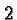, and its imaginary part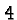. The number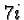is imaginary, the number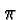is real. Both numbers are complex. In fact, all real numbers and all imaginary numbers are complex. On the other hand, some complex numbers are real, some are imaginary, and some are neither.

(A small aside: The textbook defines a complex number to be imaginary if its imaginary part is non-zero. According to that definition an imaginary number may have a non-zero real part. For example, the number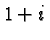would be imaginary. That definition is non-standard. I expect it's just a mistake. In any case you should not adopt that definition, it is not useful.)

The fact that some numbers are imaginary does not mean that they are inferior or mysterious, or somehow less than real. It just means they are complex numbers whose real part is zero. The word real in this context emerged in the course of history, but its modern meaning in our context is quite different from the meaning of "realistic". As far as a mathematician is concerned, real, imaginary, and complex numbers are all equally "realistic".

Here is one of the principles that make understanding mathematics possible:

You work with complex numbers as you would with ordinary algebraic expressions containing the variable, except that you replace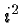with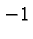wherever it occurs.

Let's look at the four basic operations. For the sake of illustration we'll combine the complex numbersand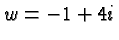. We'll also give the general formulas but you don't have to memorize them since the principle given in the previous paragraph tells you (almost) everything you need to know.

### Addition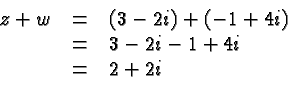In general we have that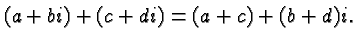### Subtraction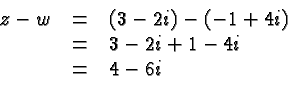In general we have that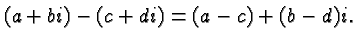### Multiplication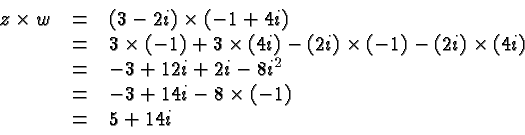In general we have that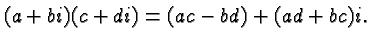That is a formula I definitely do not memorize, and you shouldn't burden your mind with it. Instead, when you multiply two complex numbers just figure out the product from the principle listed above.

### Division

Division is a little more complicated. We have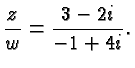If you think about it, it's not even clear that that expression is a complex number. To see that in fact it is, and to figure out its standard form, we need the concept of a conjugate complex of a complex number. It is obtained by replacing the imaginary part with its negative. Conjugate complex numbers are usually denoted with a bar. For example, the conjugate complex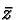ofis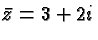and the conjugate complex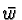of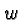is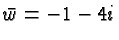. In general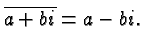The significance of the complex of a conjugate complex number is that the product of a complex number and its conjugate complex is real! For example,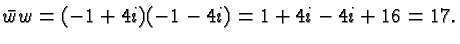In general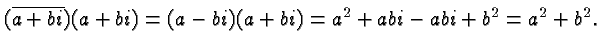This fact is so important that I'll restate it: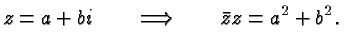We now return to the problem of dividing two complex numbers. We are bothered by the complex number in the denominator and we get rid of it by multiplying with its conjugate complex in the numerator and denominator. Hence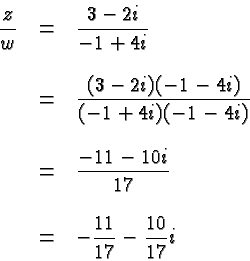The real part of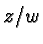is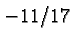and its imaginary part is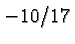. When I first saw this trick some time ago I was awed! Surely you will have something to talk about at your next party!

In general we have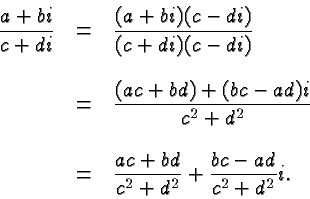That is another formula you don't want to memorize! But you should be easily able to carry out a complex division by multiplying numerator and denominator with the conjugate complex of the denominator.